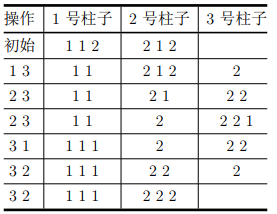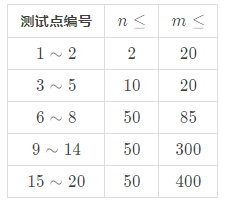## 题目描述

小 C 正在玩一个移球游戏，他面前有 $n+1$ 根柱子，柱子从 $1\sim n+1$ 编号，其中 $1$ 号柱子、$2$ 号柱子、...、$n$ 号柱子上各有 $m$ 个球，它们自底向上放置在柱子上，$n+1$ 号柱子上初始时没有球。这 $n \times m$ 个球共有 $n$ 种颜色，每种颜色的球各 $m$ 个。

1. $x$ 号柱子上至少有一个球；
2. $y$ 号柱子上至多有 $m-1$ 个球；
3. 只能将 $x$ 号柱子最上方的球移到 $y$ 号柱子的最上方。

## 输出

$0 \leq k \leq 820000$。

## 样例输入输出

$2 3 1 1 2 2 1 2$

$6 1 3 2 3 2 3 3 1 3 2 3 2$

## 提示

【样例 1 解释】【数据范围】【校验器】

checker 的使用方式为：checker <iuputfile> <outputfile>，参数依次表示输入文件与你的输出文件。

1. A x，表示进行到第 x 个操作时不合法。
2. B x，表示操作执行完毕后第 x 个柱子上的球不合法。

14

4

29%

1 秒

512 MB

NOIP2020